# AP Physics B : Understanding Gravity and Gravitational Forces

## Example Questions

### Example Question #3 : Universal Gravitation

The moon's distance from the center of the Earth was decreased by a multiple of three. How would this affect the gravitational force of the Earth on the moon?

It would increase by a factor of three

It would decrease by a factor of nine

It would increase by a factor of nine

The gravitational force would not be affected, because the moon's mass is the same

It would increase by a factor of nine

Explanation:

The law of gravitation is written as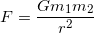, with G being equal to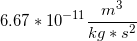.

Since the radius of the two masses acting on each other is squared, and is found in the denominator, a decrease in the radius by a multiple of three will cause a nine-fold increase in the gravitational force.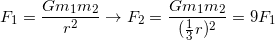### Example Question #1 : Understanding Gravity And Gravitational Forces

What is the acceleration due to gravity on a planet on which an object with a mass of 20.0kg has a weight of 270N?

27m/s2

13.5m/s2

0.074m/s2

10m/s 2

13.5m/s2

Explanation:

Solve the following equation for acceleration, using the values given in the question.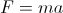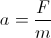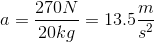### Example Question #8 : Motion In One DimensionA ball is thrown horizontally off a cliff of height of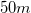with an initial velocity of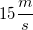. How far from the cliff will the ball land?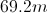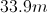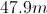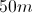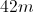Explanation:

First we will find the time required for the ball to reach the ground. Since the ball is thrown horizontally, it has no initial vertical component. We use the following equation to solve for the total flight time: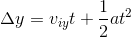We are given the change in height, initial velocity, and acceleration. Using these values, we can solve for the time. Note that the change in height will be negative, since the ball is traveling downward.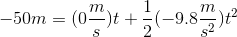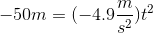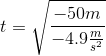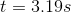Finally, we use the horizontal velocity to find the distance traveled in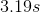. Remember that the horizontal velocity remains constant during projectile motion.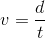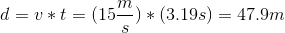### Example Question #32 : Forces

A certain planet has three times the radius of Earth and nine times the mass. How does the acceleration of gravity at the surface of this planet (ag) compare to the acceleration at the surface of Earth (g)?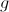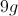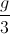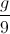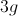Explanation:

The acceleration of gravity is given by the equation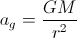, where G is constant.

For Earth,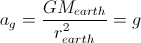.

For the new planet,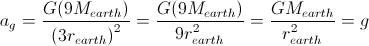.

So, the acceleration is the same in both cases.